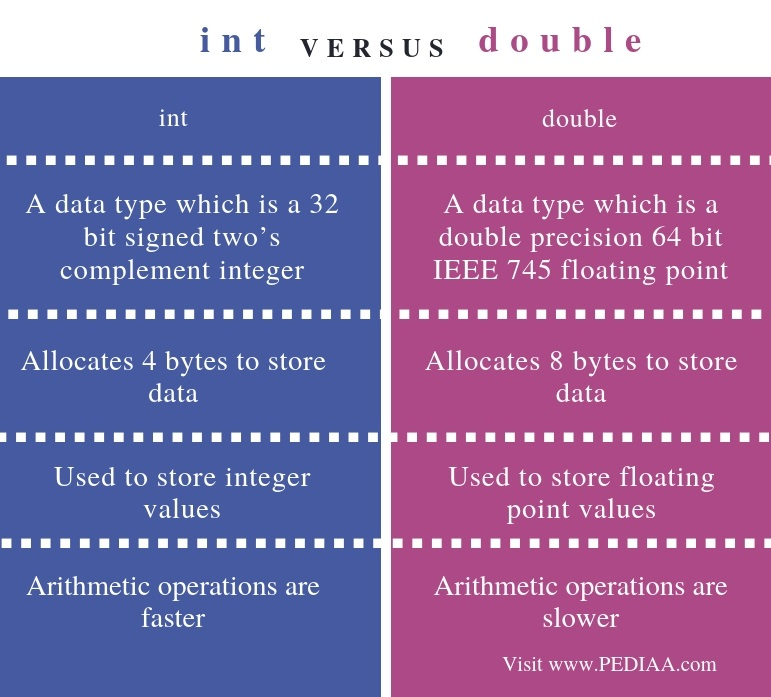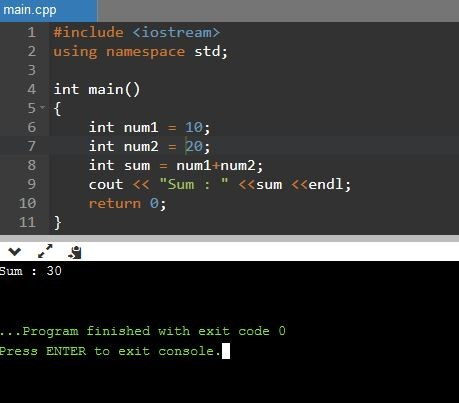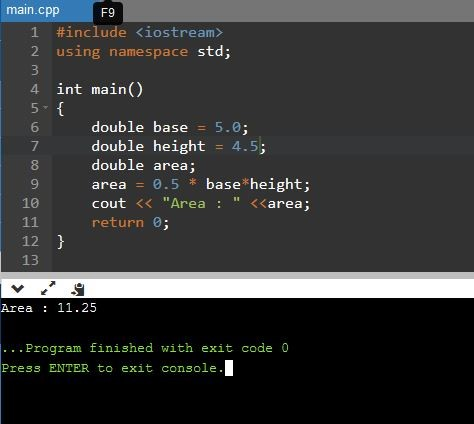What is the Difference Between int and double

The main difference between int and double is that int is used to store 32 bit two’s complement integer while double is used to store 64 bit double precision floating point value.

In programming languages such as C++, we use variables. A variable is a name given to a location that stores data. Each variable has a data type it can store. In other words, each variable can store data of a specific type. For example, a variable declared as int can only store an integer value whereas a variable declared as double can only store a double value. The data type defines how much memory it can allocate for storing a value at that memory location. The int and double are two main primitive data types. Usually, int allocates 4 bytes for data whereas double allocates 8 bytes for data.

Key Areas Covered

1. What is int
– Definition, Functionality
2. What is double
– Definition, Functionality
3. Difference Between int and double
– Comparison of Key Differences

Key Terms

int, double, VariablesWhat is int

One main primitive data type is ‘int’. The amount of memory allocated to int data type depends on the system, but the usual amount is 4 bytes.Figure 1: C++ program with int

In the above program, num1 and num2 are two variables of type int. The num1 stores 10 while num2 stores 20. The sum is another variable of type int. The total value of num1 and num2 is assigned to the sum variable. Finally, the cout statement displays the sum on the console.

What is double

Another main data type is double. The amount of memory allocated for a double depends on the system. However, usually, double allocates 8 bytes to store data.Figure 2: C++ program with double

In the above program, base and height are two variables of type double. The base variable stores 5.0 while height variable stores 4.5. The area is another variable of type double. The value calculated using base and height is assigned to the area variable. Finally, the cout statement displays the area on the console.

Difference Between int and double

Definition

While int is a data type which is a 32 bit signed two’s complement integer, double is a data type which is a double precision 64 bit IEEE 745 floating point.

Memory allocation

Memory allocation is the main difference between int and double. While int data type allocates 4 bytes to store data, double data type allocates 8 bytes to store data.

Usage

Also, another difference between int and double is that int data type is used to store integer values while the double data type is used to store floating point values.

Speed

Moreover, arithmetic operations of int are faster than double.

Conclusion

The int and double are major primitive data types. The main difference between int and double is that int is used to store 32 bit two’s complement integer while double is used to store 64 bit double precision floating point value. In brief, double takes twice memory space than int to store data.

References:

1.“C Data Types.” GeeksforGeeks, 15 Nov. 2018, Available here.About the Author: Lithmee

Lithmee holds a Bachelor of Science degree in Computer Systems Engineering and is reading for her Master’s degree in Computer Science. She is passionate about sharing her knowldge in the areas of programming, data science, and computer systems.